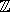# Chapter 6Modular Arithmetic

Michael Hemmer

## 6.1   Introduction

Modular arithmetic is a fundamental tool in modern algebra systems. In conjunction with the Chinese remainder theorem it serves as the workhorse in several algorithms computing the gcd, resultant etc. Moreover, it can serve as a very efficient filter, since it is often possible to exclude that some value is zero by computing its modular correspondent with respect to one prime only.

First of all, this package introduces a type CGAL::Residue. It represents/pfor some prime p. The prime number p is stored in a static member variable. The class provides static member functions to change this value. Note that changing the prime invalidates already existing objects of this type. However, already existing objects do not lose their value with respect to the old prime and can be reused after restoring the old prime. Since the type is based on double arithmetic the prime is restricted to values less than 226. The initial value of p is 67111067.

Please note that the implementation of class CGAL::Residue requires a mantissa precision according to the IEEE Standard for Floating-Point Arithmetic (IEEE 754). However, on some processors the traditional FPU uses an extended precision. Hence, it is indispensable that the proper mantissa length is enforced before performing any arithmetic operations. Moreover, it is required that numbers are rounded to the next nearest value. This can be ensured using CGAL::Protect_FPU_rounding with CGAL_FE_TONEAREST, which also enforces the required precision as a side effect.advancedIn case the flag CGAL_HAS_THREADS is undefined the prime is just stored in a static member of the class, that is, CGAL::Residue is not thread-safe in this case. In case CGAL_HAS_THREADS the implementation of the class is thread safe using boost::thread_specific_ptr. However, this may cause some performance penalty. Hence, it may be advisable to configure CGAL with CGAL_HAS_NO_THREADS.advancedMoreover, the package introduces the concept Modularizable. An algebraic structure T is considered as Modularizable if there is a mapping from T into an algebraic structure that is based on the type CGAL::Residue. For scalar types, e.g. Integers, this mapping is just the canonical homomorphism into/prepresented by CGAL::Residue. For compound types, e.g. Polynomials, the mapping is applied to the coefficients of the compound type. The mapping is provided by the class CGAL::Modular_traits<T>. The class CGAL::Modular_traits<T> is designed such that the concept Modularizable can be considered as optional, i.e., CGAL::Modular_traits<T> provides a tag that can be used for dispatching.

### 6.1.1   Example

In the following example modular arithmetic is used as a filter.

```File: examples/Modular_arithmetic/modular_filter.cpp
```
```/* Modular arithmetic can be used as a filter, in this example modular
arithmetic is used to avoid unnecessary gcd computations of polynomials.
A gcd computation can be very costly due to coefficient growth within the
Euclidean algorithm.

The general idea is that firstly the gcd is computed with respect
to one prime only. If this modular gcd is constant we can (in most cases)
conclude that the actual gcd is constant as well.

For this purpose the example introduces the function may_have_common_factor.
Note that there are two versions of this function, namely for the case
that the coefficient type is Modularizable and that it is not.
If the type is not Modularizable the filter is just not applied and the
function returns true.
*/

#include <CGAL/basic.h>

#ifdef CGAL_USE_GMP

#include <CGAL/Gmpz.h>
#include <CGAL/Polynomial.h>

// Function in case  Polynomial is Modularizable
template< typename Polynomial >
bool may_have_common_factor(
const Polynomial& p1, const Polynomial& p2, CGAL::Tag_true){
std::cout<< "The type is modularizable" << std::endl;

// Enforce IEEE double precision and rounding mode to nearest
// before useing modular arithmetic
CGAL::Protect_FPU_rounding<true> pfr(CGAL_FE_TONEAREST);

// Use Modular_traits to convert to polynomials with modular coefficients
typedef CGAL::Modular_traits<Polynomial> MT;
typedef typename MT::Residue_type MPolynomial;
typedef typename MT::Modular_image Modular_image;
MPolynomial mp1 = Modular_image()(p1);
MPolynomial mp2 = Modular_image()(p2);

// check for unlucky primes, the polynomials should not lose a degree
typename CGAL::Polynomial_traits_d<Polynomial>::Degree  degree;
typename CGAL::Polynomial_traits_d<MPolynomial>::Degree mdegree;
if ( degree(p1) != mdegree(mp1)) return true;
if ( degree(p2) != mdegree(mp2)) return true;

// compute gcd for modular images
MPolynomial mg  = CGAL::gcd(mp1,mp2);

// if the modular gcd is not trivial: return true
if ( mdegree(mg) > 0 ){
std::cout << "The gcd may be non trivial" << std::endl;
return true;
}else{
std::cout << "The gcd is trivial" << std::endl;
return false;
}
}

// This function returns true, since the filter is not applicable
template< typename Polynomial >
bool may_have_common_factor(
const Polynomial&, const Polynomial&, CGAL::Tag_false){
std::cout<< "The type is not modularizable" << std::endl;
return true;
}

template< typename Polynomial >
Polynomial modular_filtered_gcd(const Polynomial& p1, const Polynomial& p2){
typedef CGAL::Modular_traits<Polynomial> MT;
typedef typename MT::Is_modularizable Is_modularizable;

// Try to avoid actual gcd computation
if (may_have_common_factor(p1,p2, Is_modularizable())){
// Compute gcd, since the filter indicates a common factor
return CGAL::gcd(p1,p2);
}else{
typename CGAL::Polynomial_traits_d<Polynomial>::Univariate_content  content;
return CGAL::gcd(content(p1),content(p2)); // return trivial gcd
}
}

int main(){
CGAL::set_pretty_mode(std::cout);

typedef CGAL::Gmpz NT;
typedef CGAL::Polynomial<NT> Poly;

Poly  f1(NT(2), NT(6), NT(4));
Poly  f2(NT(12), NT(4), NT(8));
Poly  f3(NT(3), NT(4));

std::cout << "f1        : " << f1 << std::endl;
std::cout << "f2        : " << f2 << std::endl;

std::cout << "compute modular filtered gcd(f1,f2): " << std::endl;
Poly g1 = modular_filtered_gcd(f1,f2);
std::cout << "gcd(f1,f2): " << g1 << std::endl;

std::cout << std::endl;
Poly p1 = f1*f3;
Poly p2 = f2*f3;

std::cout << "f3        : " << f3 << std::endl;
std::cout << "p1=f1*f3  : " << p1 << std::endl;
std::cout << "p2=f2*f3  : " << p2 << std::endl;

std::cout << "compute modular filtered gcd(p1,p2): " << std::endl;
Poly g2 = modular_filtered_gcd(p1,p2);
std::cout << "gcd(p1,p2): " << g2 << std::endl;
}

#else

int main (){
std::cout << " This examples needs GMP! " << std::endl;
}

#endif
```

## 6.2   Design and Implementation History

The class CGAL::Residue is based on the C-code of Sylvain Pion et. al. as it was presented in [BEPP99].

The remaining part of the package is the result of the integration process of the NumeriX library of EXACUS [BEH+05] into CGAL.